# GED Math : Decimals and Fractions

## Example Questions

1 2 3 4 6 Next →

### Example Question #51 : Decimals And Fractions

Findof 88.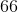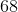Explanation:

To find a fraction of a whole number, we will multiply the two numbers together. So, we get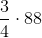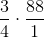Now, we can simplify. The 4 and the 88 can both be divided by 4. So, we get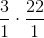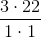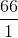Therefore,of 88 is 66.

### Example Question #52 : Decimals And Fractions

What isof?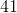Explanation:

To find a fraction of a whole number, we will multiply the two together. So, we get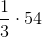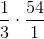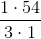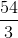Therefore,ofis.

### Example Question #53 : Decimals And Fractions

Which of the following fractions must equal to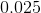?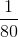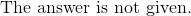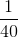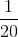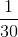Explanation:

To convert the decimal into a fraction, we can set up a proportion such that some number out of 100 is equal to the decimal.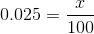Multiply by 100 on both sides.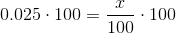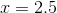The fraction we will need to reduce is: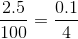To eliminate the decimal we can move the decimal place to the right one place on the top and bottom.

The answer is:### Example Question #54 : Decimals And Fractions

One pan of Super Chocolate brownies requires one and one third cups of sugar and one and one fourth cups of chocolate chips. Brenda wants to make eight pans of Super Chocolate brownies. She has ten cups of sugar and ten cups of flour on hand. Does she have enough sugar and flour to make these brownies?

Brenda has enough sugar but not enough flour.

Brenda has enough of both ingredients.

Brenda has enough flour but not enough sugar.

Brenda does not have enough of either ingredient.

Brenda has enough flour but not enough sugar.

Explanation:

Multiply the number of cups of sugar per batch,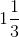, by the number of batches Brenda wants to make, 8.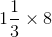Rewrite both numbers as improper fractions as follows: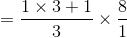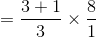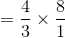Multiply the fractions by multiplying numerators, then denominators: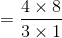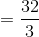Rewrite as a mixed number by dividing the numerator by the denominator: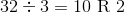, so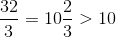Brenda needs more than the ten cups of sugar she has on hand.

Perform the same steps with flour. Since Brenda needs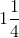cups per batch: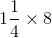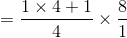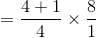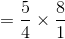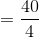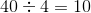, soBrenda needs 10 cups of flour, the exact amount she has on hand.

Brenda has enough flour but not enough sugar.

### Example Question #54 : Decimals And Fractions

4.188, 3.8, 2.55, 3.19

Multiply this sum by 6.

Round this result to the nearest whole number.

What is that number?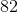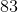Explanation:

Add the decimal expressions as you would integers: append placeholder zeroes so that each number has the same number of decimal places, line up decimal points; add, (temporarily) disregarding the decimal points, and, in the sum, line up the decimal point with those in the addends. The result: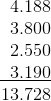Multiply by 6 as you would integers, again (temporarily) disregarding the decimal point; place the decimal point in the product so that three digits are to the right (the total number in the factors):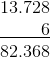Round this to the nearest whole number by noting that the next digit to the right (the tenths digit) is 3; therefore, round down to 82, the correct response.

### Example Question #56 : Decimals And Fractions

Peter ran each day during a five-day period. The number of miles he ran on each day was as follows:

Monday: One mile.

Tuesday: One and one half miles.

Wednesday: One mile.

Thursday: Two miles.

Friday: One half mile.

Give the total number of miles Peter ran per day over the five-day period in question.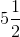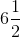Explanation:

The sum of the numbers of miles Peter ran is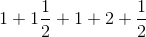Rewrite the expressions in terms of halves, and add numerators: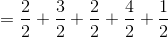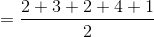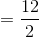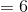This is the correct response.

### Example Question #51 : Decimals And Fractions

Simplify the following expression: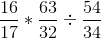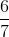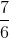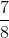Explanation:

Simplify the following expression:To begin, let's recall that dividing by a fraction is the same thing s multiplying by its reciprocal.

Therefore our original expression becomes: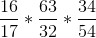Now, we should also recall that when multiplying fractions, we simply multiply across the top and the bottom to get our answer. This means we can combine these three fractions into one big fraction.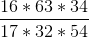Now, we could just go to our calculator and plug this in and multiply it all out, but first let's simplify by removing common factors on the top and bottom.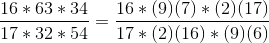Now, cancel out those factors found in both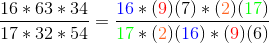Those pairs of numbers will cancel to yield:1 2 3 4 6 Next →